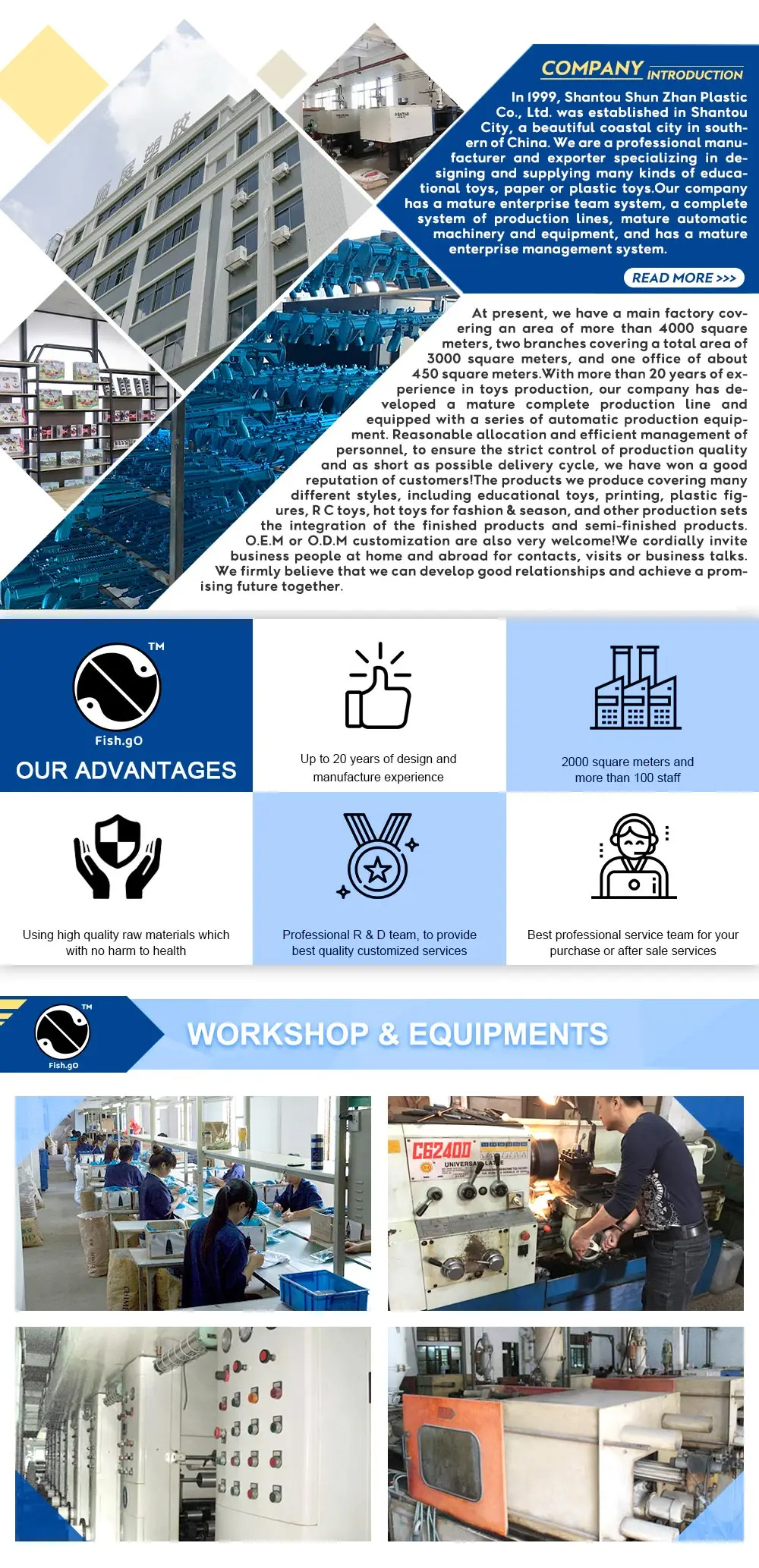?

?

Packaging & Shipping

?

 Color box Box Style Weightproduct&box SETS/CTN Measure G.W / N.W. 27.5*20.5*5.5 Heaven and earth cover 781g 18 48x59x26.5cm 14 / 16 kg

?

?

Company Information

??

FAQ

?(function(e){if(!e["_med"])e["_med"]={};var t=e["_med"];t.cookie=function(e,t,a){if(t!==undefined){a=a||{};if(typeof a.expires==="number"){var o=a.expires,l=a.expires=new Date;l.setTime(+l+o*864e5)}return document.cookie=[e,"=",String(t),a.expires?"; expires="+a.expires.toUTCString():"",a.path?"; path="+a.path:"",a.domain?"; domain="+a.domain:"",a.secure?"; secure":""].join("")}var r=e?undefined:{};var i=document.cookie?document.cookie.split("; "):[];for(var n=0,s=i.length;n<s;n++){var b=i[n].split("=");var d=b.shift();var p=b.join("=");if(e&&e===d){r=p;break}if(!e&&p!==undefined){r[d]=p}}return r};var a=document;var o=e.devicePixelRatio||1,l=a.documentElement.clientWidth,r=a.documentElement.clientHeight,i,n,s,b=/initial-scale=([\d\.]+?),/i,d,p;if(a.querySelector){p=a.querySelector('meta[name="viewport"]');if(p){d=b.exec(p.content+",");if(d){s=parseFloat(d,10)}}}if(s){l=l*s;r=r*s}if(screen){if(Math.abs(screen.width-l*o)<.2*screen.width){l=screen.width/o;r=screen.height/o;i=screen.width;n=screen.height}else{l=screen.width;r=screen.height;i=screen.width*o;n=screen.height*o}}else{i=l*o;n=r*o}var m="createTouch"in a&&"ontouchstart"in e?1:0;var c=["dw:"+l,"dh:"+r,"pw:"+i,"ph:"+n,"ist:"+m].join("&");t.cookie("_med",c,{expires:3650})})(window); 2020最新无码福利视频,欧美日韩在线亚洲综合国产人,亚洲A片无码2019
<strike id="c7fri"></strike>
<strike id="c7fri"></strike>